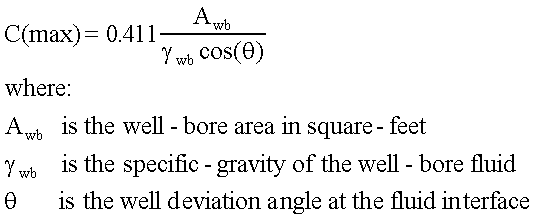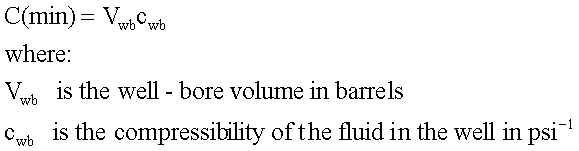## ESTIMATE THE STORAGE-COEFFICIENT

The next piece of information required is an estimate of the possible range of the well-bore storage-coefficient. This coefficient measures the volume of fluid that enters the well-bore per unit-change in the bottom-hole pressure. Because this is a well-bore coefficient, it can be estimated strictly from the well-bore geometry and the fluid in the well-bore.

FIRST: calculate the MAXIMUM storage-coefficient, C(max). This value corresponds to a free fluid interface in the well-bore and a well-head pressure of zero.NEXT: calculate the MINIMUM storage-coefficient, C(min). This value corresponds to a fluid-filled well-bore.Calculate C(min) and C(max) and write down the values you obtain; we need them later!

Q: Did you calculate C(min) and C(max) and write down the values?